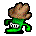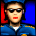# MPL115A2 temperature measurement?

cancel
Showing results for
Did you mean:

## MPL115A2 temperature measurement?

2,222 ViewsContributor I

Hi Guys,
Does anybody know if it is possible to get accurate temperature form data delivered by the sensor. I tried to use following equation:

Temp_comp = (605.75 - Temp_ADC) / 5.35

but calculated temperature was smaller than it should be. Any ideas? Best regards... Robert

3 Replies
760 ViewsContributor I

I know this post is from last year.

But I have the same problem.

According to the Datasheet the Temperature-ADC-Value is 472 at 25°C. While each degree counts -5.35.

So if I use " Temp = (ADC_val - 472)/-5.35 +25"

I got "11" at 21°C. So if I use "Temp = (ADC_val - 472)/-5.35 +35 " I got the right value.

So I think theres a mistake in the Datasheet (and Application Note AN3785 too). Because "472" @25°C can't be right. I have something about 520-530

760 ViewsContributor I

I had the same problem of figuring out how to convert Tadc to actual degrees.

This is the response from Freescale:

"Anyway, while we output temp on MPL115A, the temp sensor is used to calibrate and make our Pressure output more accurate, not provide accurate temperature determination. That was a cost reduction choice. If we provided a calibrated temperature, then we would add cost to the test procedure. The next generation MPL3115A now has calibrated temp output. Its production is is end of Q4 this year.

Regarding temperature conversion, the fact is that the 25 deg C is supposed to be set at 472 ADC counts on the Tadc output from MPL115A. It is a negative slope, such that its -5.35 ADC counts per a deg C. I worked out the transfer function to be somewhat like:

Temp = (Tadc - 605.75) / -5.35

At -40 deg C the output value should be of 819.75 and at 105 deg C the output value should be of 44.

Note that because the value is a 10 bit, it cannot in the real world always be assumed to be a full range output. Even a 9 bit number cannot accommodate this range, so its 10. "

760 ViewsContributor I

Ok this helps a little bit, but it does not explain why the adc-count at 25°C is 530 instead of 472. So the temperature differs about 10 degree. Isn't this a too big variance, even for a uncalibrated temperature-sensor?